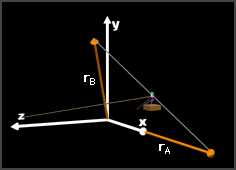Ch 2. Vectors Multimedia Engineering Statics Scalars & Vectors 2-DVectors 3-DVectors DotProducts
 Chapter 1. Basics 2. Vectors 3. Forces 4. Moments 5. Rigid Bodies 6. Structures 7. Centroids/Inertia 8. Internal Loads 9. Friction 10. Work & Energy Appendix Basic Math Units Sections Search eBooks Dynamics Fluids Math Mechanics Statics Thermodynamics Author(s): Kurt Gramoll ©Kurt GramollSTATICS - CASE STUDY IntroductionConstruction Trolley SystemDisplacement Vectors Some workers are refurbishing the Eiffel Tower. The construction crew utilizes a trolley system to transport equipment from point A on the ground to point B on the platform they are working on. The trolley is pulled by two cables at the base of the tower. What is known: With an axis system oriented as shown, the position vectors of points A and B are rA = 175i + 0j + 0k m rB = 39i + 70j + 29k m When the trolley is halfway between points A and B, the forces exerted on the trolley by the cables are F1 = -943.7i - 221.7j + 245.4k m F2 = -919.4i - 216.0j - 328.6k m Question What is the component of the force exerted on the trolley that is parallel to the line AB? Approach Find the unit vector in the direction of the line AB. Find the total force exerted on the trolley by the two cables. Solve for the component of the total force that is parallel to the unit vector for line AB.

Practice Homework and Test problems now available in the 'Eng Statics' mobile app
Includes over 500 problems with complete detailed solutions.
Available now at the Google Play Store and Apple App Store.